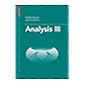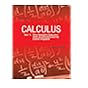Normal view MARC view ISBD view

# Calculus, vol. II Multi-Variable Calculus and LInear Algebra, with Applications to Differential Equations and Probability

Material type:TextPublisher: 1969Edition: 2 nd. ed.Description: 673 p. illus. 27 cm.ISBN: 0471000086.Subject(s): Mathematical analysis | CalculusSummary: (Acc. No. 1901) Instructors Copy
List(s) this item appears in: Reserved for the semester Aug-Nov 2017
Item type Current location Call number Status Date due Barcode Item holds
Book Chennai Mathematical Institute
General Stacks
515 APO (Browse shelf) Available 2227
Book Chennai Mathematical Institute
515 APO (Browse shelf) Available 1901
Book Chennai Mathematical Institute
515 APO (Browse shelf) Available 1745
Book Chennai Mathematical Institute
515 APO (Browse shelf) Available 2228
Book Chennai Mathematical Institute
515 APO (Browse shelf) Available 1750
Total holds: 0
##### Browsing Chennai Mathematical Institute Shelves , Shelving location: General Stacks Close shelf browser
 No cover image available No cover image available515 AMA Analysis II / 515 AMA Analysis III / 515 APO Calculus, vol. I One-Variable Calculus, with an introduction to linear algebra 515 APO Calculus, vol. II Multi-Variable Calculus and LInear Algebra, with Applications to Differential Equations and Probability 515 APO Calculus / Vol. 1 one variable calculus, with an introduction to linear algebra. 515 APO Calculus / Vol. 1 one variable calculus, with an introduction to linear algebra. 515 APO Calculus / Vol. 1 one variable calculus, with an introduction to linear algebra.

(Acc. No. 1901) Instructors Copy